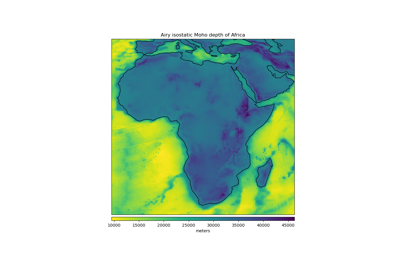# harmonica.isostasy_airy¶

harmonica.isostasy_airy(topography, density_crust=2800.0, density_mantle=3300.0, density_water=1000.0, reference_depth=30000.0)[source]

Calculate the isostatic Moho depth from topography using Airy’s hypothesis.

According to the Airy hypothesis of isostasy, topography above sea level is supported by a thickening of the crust (a root) while oceanic basins are supported by a thinning of the crust (an anti-root). This assumption is usuallySchematic of isostatic compensation following the Airy hypothesis.

The relationship between the topographic/bathymetric heights ($$h$$) and the root thickness ($$r$$) is governed by mass balance relations and can be found in classic textbooks like [TurcotteSchubert2014] and [Hofmann-WellenhofMoritz2006].

On the continents (positive topographic heights):

$r = \frac{\rho_{c}}{\rho_m - \rho_{c}} h$

while on the oceans (negative topographic heights):

$r = \frac{\rho_{c} - \rho_w}{\rho_m - \rho_{c}} h$

in which $$h$$ is the topography/bathymetry, $$\rho_m$$ is the density of the mantle, $$\rho_w$$ is the density of the water, and $$\rho_{c}$$ is the density of the crust.

The computed root thicknesses will be added to the given reference Moho depth ($$H$$) to arrive at the isostatic Moho depth. Use reference_depth=0 if you want the values of the root thicknesses instead.

Parameters
• topography (array or xarray.DataArray) – Topography height and bathymetry depth in meters. It is usually prudent to use floating point values instead of integers to avoid integer division errors.

• density_crust (float) – Density of the crust in $$kg/m^3$$.

• density_mantle (float) – Mantle density in $$kg/m^3$$.

• density_water (float) – Water density in $$kg/m^3$$.

• reference_depth (float) – The reference Moho depth ($$H$$) in meters.

Returns

moho_depth (array or xarray.DataArray) – The isostatic Moho depth in meters.

## Examples using harmonica.isostasy_airy¶